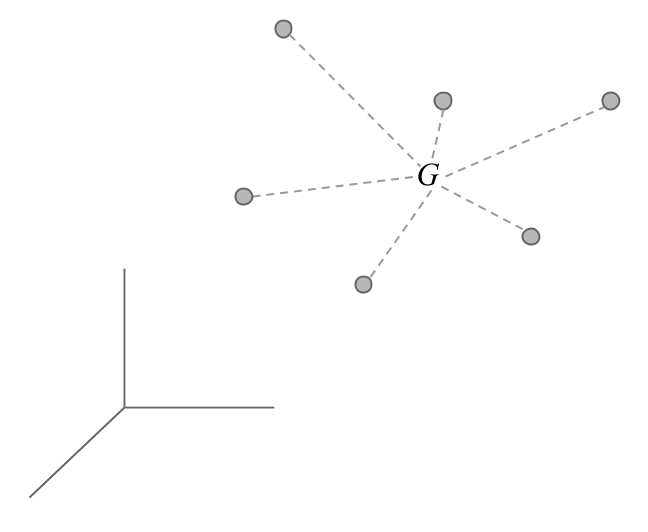5.3 Center of Gravity

For every cloud of points, there is an average point, called the center of gravity.If the points have an associated weight ($$p_i$$ for individual $$i$$), the coordinate of the center of gravity on the $$i$$ axis is:

$G_j = \sum_{i=1}^{n} p_i \hspace{1mm} x_{ij}$

The center of gravity corresponds the statistical notion of mean or average point.